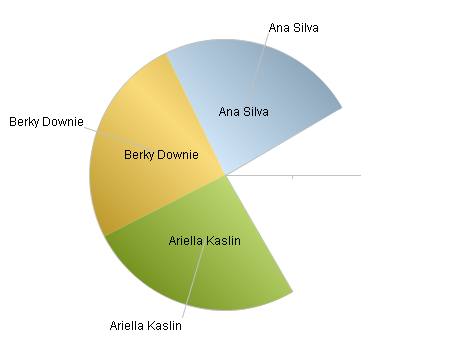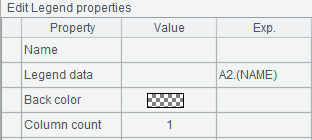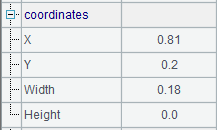# 环图元

## 环图元及其数据属性

 A 1 =canvas() 2 =demo.query("select * from GYMSCORE where Event = 'Vault'") 3 =A1.plot("BackGround") 4 =A1.plot("EnumAxis","name":"x","location":3,"polarX":0.55, "allowLabels":false) 5 =A1.plot("NumericAxis","name":"y","location":4,"allowLabels":false) 6 =A1.plot("Sector","axis1":"x","data1":A2.(NAME),"axis2":"y", "data2":A2.(SCORE),"text":A2.(NAME)) 7 =A1.draw@p(400,300)

A1中建立画布。A2中获取绘图数据如下：A3中绘制背景，A4中绘制枚举轴作为极轴xA5中绘制数值轴作为角轴y。特别的，横轴和纵轴的Allow Labels属性均设为了false，不绘制极坐标的刻度，以防止其干扰饼图。

A6中用环图元绘制饼图，这是我们在这里主要研究的图元。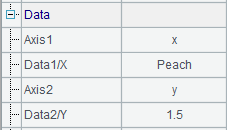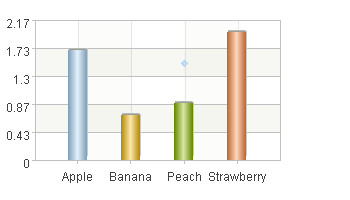## 环图元的外观属性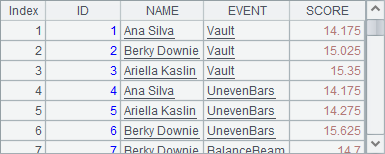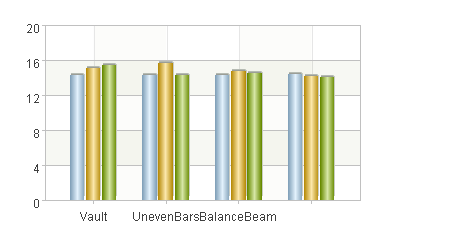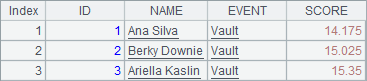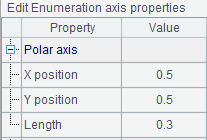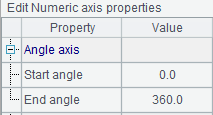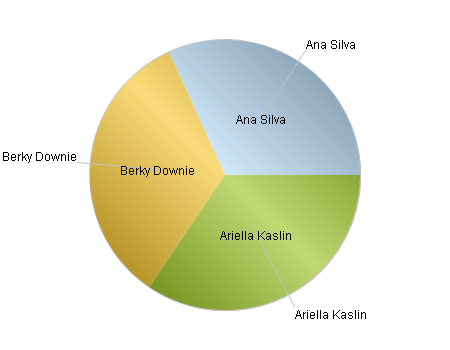## 多系列饼图

 A 1 =canvas() 2 =demo.query("select * from GYMSCORE order by NAME,EVENT") 3 =A1.plot("BackGround") 4 =A1.plot("EnumAxis","name":"x","location":3,"polarX":0.55,"polarY": 0.4,"allowLabels":false) 5 =A1.plot("NumericAxis","name":"y","location":4,"allowLabels":false) 6 =A1.plot("Sector","axis1":"x","data1":A2.(NAME+","+EVENT),"axis2": "y","data2":A2.(SCORE)) 7 =A1.plot("Legend","legendText":A2.id(EVENT),"columns":2,"x":0.2,"y":0.8) 8 =A1.draw@p(400,400)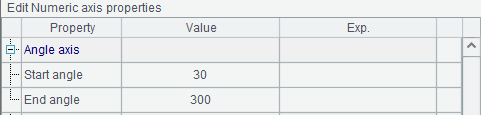A8的绘图结果如下：